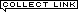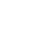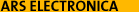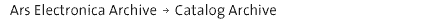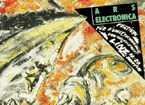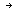Ars Electronica 1986Festival-Program 1986Back to:Festival 1979-2007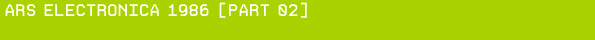Les petites Histories de Mamy Computer 'Hervé Huitric Hervé Huitric / 'Monique Nahas Monique Nahas INTRODUCTIONArtists who want to develop personalized computer tools to create images must choose among varying modes of object representation. Painting and animation techniques that they might wish to construct for coloring, shading, texturizing, or transforming surfaces will depend on the type of representation chosen.We decided to use B-spline surfaces for several reasons: first, to satisfy our personal taste–preference for smooth surfaces and continuous variations in form as well as color- second, because the Oslo algorithm provides a sculpturing tool for which we believe there is no equivalent- finally, because the calculation time that once constituted an inconvenience is now becoming quite reasonable.USE OF B-SPLINES IN GENERATING 3–D IMAGESThree-dimensional images can be obtained using B-splines whose control points are defined by three coordinates, X, Y, Z. Hidden parts and shadows are calculated by Z-buffer and S-buffer techniques that we described in "Computer Art with Rodin". All the points of an image are not calculated, since only a set of points whose density depends on the complexity of the surface need to be calculated. The surface is then filled in one of the following ways:The points are interpolated bilinearly, four by four, producing automatic Gouraud shading of the surface.The points can be interpolated linearly between two consecutive points, thereby generating a network of curves for U and V of the surface similar to those in the laced hand in Figure 1. This process produces a mode of fiber representations that we've used to generate images of forests and hair, for instance.The points are not at all interpolated, creating a pointillistic surface. In this case the density of calculated points is a parameter that permits the construction of surfaces with varying densities, as in Figure 2.Y-A-PAS-EU-D'ADAMThis historical sequence utilize different technicsThe interpolation of control points.The Fiber-modeAn evolutive technic of texture.CONTROL POINT INTERPOLATION. By interpolating the control points of two B-spline surfaces, we obtain a 3-D interpolation. The Oslo algorithm is very useful here, too, as it allows us to equalize the number of control points of the surfaces to be interpolated and to introduce new control points in selected places. Two sets of control points can thus be transformed into a new set by a blending function:F (P1i,j, P2i,j) = Pi,jAll functions F that are continuous with respect to their parameters and variables will produce continuous sequences. One example is the linear interpolationFn(P1i,j, P2i,j) = ai,j(n)•P1i,j + (1– ai,j(n))•P2i,jThis example shows an interpolation between objects 1 and 2 when coefficients i and j are equal to 1 before the transformation and equal to 0 at the end. If these coefficients depend on the values of i and j, mixed images will result at each stage of the transformation. The body rising from the water in this sequence is an example of this kind of interpolation.UN JEU D'ENFANTThis sequence mixes two male fantasies, cars and the female body.We utilize a 3–D modulation to accentuate the movement of the car during the animation.DU CUL, DU CUL, RIEN QUE DU CULThis sequence is meaningless by principle. Technically the part where Satyrs do what they must do is interesting.We consider it interesting to investigate global transformations that bring into account relationships between control points. Through discrete Fourier transformations of control points, a matrix of coefficients reflecting the linear relationship between control points is obtained. A continuous transformation of these coefficients produces a continuous deformation of the image by calculating new control points of the inverse Fourier transformation.For example, we used both high and low pass filters on the Fourier coefficients of the heads. The effects that produced a smirk were obtained by using high-pass filters applied continuously.GAME OVERThis sequence simulates the reflection of a body on B-spline walls. Each frame needs 4 hours of computation on a Vax 780.We simulate reflection and refraction of light on B-spline surfaces by using the ray-tracing techniques described by Whiffed. We traverse the reflecting or refracting surface and for each point on it we determine the ray going from that point to the eye. Then the brightness of the point is determined by optical geometry: light can come from a ray symmetric with respect to the normal in the case of reflection, or from a ray determined by the index of refraction in the case of refraction.To find the intensity along these rays, we intersect them with the other objects of the scene. The same process is then repeated recursively at each point of intersection.We calculate the intersection of a ray with a B-spline surface using the Oslo algorithm recursively. We cut the B-spline into four sub-surfaces and determine if the ray can intersect each of the sub-surfaces. If a potential intersection with a sub-surface is detected, we subdivide again, and so on. Intersection tests are made by examining the largest cube that encloses the control points of a sub-surface. Since B-splines have the convex hull property, a ray that does not intersect an enclosing cube cannot intersect the surface itself. References:E. COHEN, T. LYCHE, AND R. RIESENFELD, "Discrete B-Splines and Subdivision Techniques in Computer Aided Geometric Design and Computer Graphics", COMPUTER GRAPHICS AND IMAGE PROCESSING, Vol. 14, No. 2, 1980, pp. 87–111.H. HUITRIC AND M. NAHAS, "Computer Art with Rodin", INFORMATION PROCESSING 83, R.E.A. Mason, ed., IFIP 1983, pp. 275–282.H. GOURAUD, "Continuous Shading of Curved Surfaces", IEEE TRANS. COMPUTERS, Vol. C–20, No. 6, June 1971, pp. 623–628.T. WHITTED, "An Improved Illumination Model for Shaded Display", COMM. ACM, Vol. 23, No. 6. June 1980, pp. 343–349.FILMS D'IMAGES SYNTETIQUEBUS 307, 16 mm, 1979, 7'BOBOS-NONOS, 16 mm, 1980, 10'BOULOUT, 16 mm, 1981, 3' 9600BANDS, 16 mm, 1982, 5'PEDAGOGIQUE, 16 mm, 1983, 15'
 © Ars Electronica Linz GmbH, info@aec.at